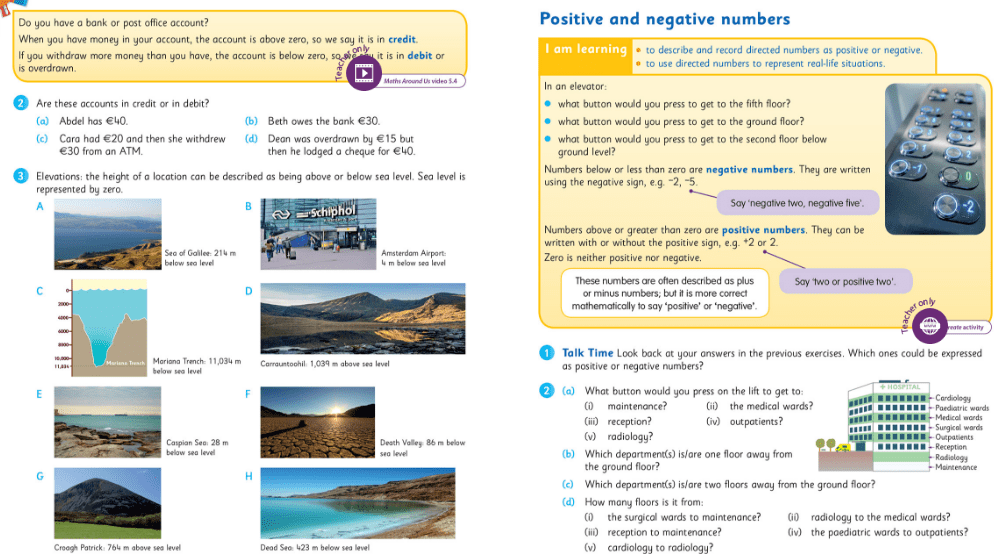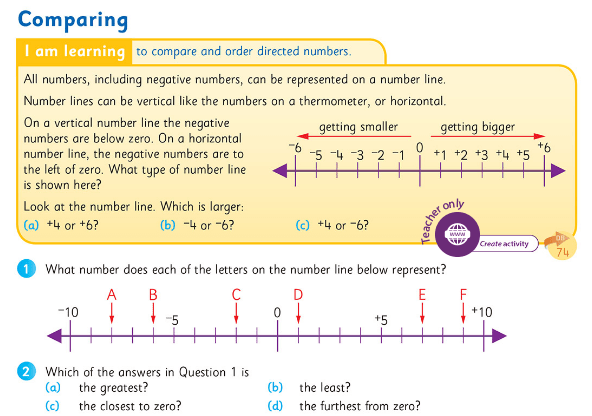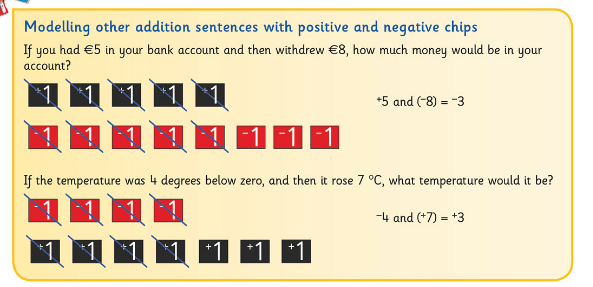# Digging Deeper into … Directed Numbers (5th & 6th classes)

## Digging Deeper into … Directed Numbers (5th & 6th classes)

Category : Uncategorized

For practical suggestions for families, and links to useful digital resources, to support children learning about the topic of area, please check out the following post: Dear Family, your Operation Maths Guide to Directed Numbers

In Operation Maths for 5th Class the children are formally introduced to the concept of directed numbers (from the strand of algebra) and are enabled to:

• Explore and identify directed numbers, i.e. numbers above and below zero, including zero
• Describe and record directed numbers as positive or negative
• Use directed numbers to represent real-life situations
• Compare and order directed numbers
• Solve problems involving directed numbers.

In Operation Maths for 6th class, this knowledge and understanding is revised and extended to include the addition of positive and negative numbers.

Directed numbers (formally known as integers, the set of positive and negative whole numbers including zero) can be a very difficult concept for some children for a number of reasons:

• Being able to explore numbers less than zero requires a solid understanding of zero, an abstract concept in itself since it indicates the absence of something
• Many children find it difficult to comprehend how something can be less than zero. Therefore, when ordering it can be difficult for many children to appreciate that 0 is greater than -1, -2, etc.
• Operations involving integers are particularly troublesome as having similar signs so close together can be very confusing.

Use real-life contexts

In fifth class, since this is the first time that the children have been formally introduced to negative numbers, it is essential that this happens through reference to real-life contexts, e.g. temperature, buttons in a lift, goal difference in soccer league tables, depths in a swimming pool, or any other context with which the children can readily identify. Other real-life contexts such as bank account balances, elevations, etc., can also be used.### Use ‘positive’ and ‘negative’ rather than ‘plus’ and ‘minus’

Whilst real-life contexts are useful, they can also be confusing, particularly when plus and minus are used to describe directed numbers. Insist that the children use the language of positive and negative, since that is more correct mathematically. Using positive and negative can also reduce complications in future classes when calculating with directed numbers, e.g. avoiding having to say three minus minus five for 3-(-5). This is particularly important when the children progress to adding positive and negative numbers: for example (–3) + (+9) should be read as ‘negative three add/plus positive nine’ rather than ‘minus 3 plus plus 9’. It is also important that the children recognise that positive numbers can be written either with, or without, the positive sign, therefore we can assume that any number without a sign is positive.

To further reduce complications with written forms of directed numbers, raised signs should be used for the positive and negative (as shown in image below) and brackets should be used to make it easier for the child to distinguish between the operations signs (i.e. + and -) and the directed numbers signs.### Representing directed numbers

Since negative numbers are so abstract, it is vital that opportunities to represent them concretely and pictorially are maximised. Many children consider only the cardinality of numbers, i.e. that they represent the number of objects in a set, whereas understanding of directed numbers relies on understanding the ordinality of numbers; this is why visual representations are so vital.

One way to do this, is to use a counting stick, if available. Start with the counting stick in a vertical position, asking the children that if the centre line is now representing zero, can they identify the points above and below that?

• Initially describe the positions as “one above zero”, “two below zero” etc
• Then, when appropriate, introduce positions as “positive three”, “negative four” etc
• Development: if each interval now represents two/ten/five as opposed to one, how does this change the identity of the positions?
• Finally, move the counting stick into a horizontal position, so that the negative numbers are now to the left of the centre/zero and the positive to the right (as the children see it).

Operation Maths users can also use the counting stick eManipulative to model the counting stick in a horizontal position, as shown below (Hint: zoom the screen in to 150% to view the numbers better).Number lines are also very useful. When using number lines (or a counting stick), emphasize that numbers get smaller in value as you move above/to the left of zero, and larger as you move below/to the right of zero . If possible, have a number line that includes negative numbers on permanent display in the classroom.

The Operation Maths MWBs can also be used to create a dynamic number line for ordering activities. For example, ask individual children to write a specific integer on their MWB. Then ask a group of children, each with different integers,  to stand out and put their MWBs in order. These numbers could even be hung from a ‘washing line’ using clothes pegs.

In sixth class, to better illustrate the processes involved with the addition of integers, teachers are encouraged to use the positive and negative chips, that accompany the sixth class books as part of the free ancillary resources (they’re on the same sheet as the images of the base ten block for modelling decimal numbers).The teacher can also use the positive and negative chips built into the Sorting eManipulative to model calculations on the class IWB, as shown below (the positive and negative chips are located at the end of the numbers tab).The children can then progress to using number lines to both reinforce their understanding of addition with integers and extend it to bigger numbers. A physical number line that the children can walk along would be ideal initially, as this suits the kinaesthetic learners and the bigger nature makes it easier for teachers to assess the children’s ability to use the number line before they move to smaller scale number lines in books and copies.